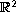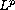Electron. J. Diff. Eqns., Vol. 2008(2008), No. 163, pp. 1-25.

### Sharp asymptotic estimates for vorticity solutions of the 2D Navier-Stokes equation Yuncheng You

Abstract:
The asymptotic dynamics of high-order temporal-spatial derivatives of the two-dimensional vorticity and velocity of an incompressible, viscous fluid flow inare studied, which is equivalent to the 2D Navier-Stokes equation. It is known that for any integrable initial vorticity, the 2D vorticity solution converges to the Oseen vortex. In this paper, sharp exterior decay estimates of the temporal-spatial derivatives of the vorticity solution are established. These estimates are then used and combined with similarity andcompactness to show the asymptotical attraction rates of temporal-spatial derivatives of generic 2D vorticity and velocity solutions by the Oseen vortices and velocity solutions respectively. The asymptotic estimates and the asymptotic attraction rates of all the derivatives obtained in this paper are independent of low or high Reynolds numbers.

Submitted November 5, 2008. Published December 18, 2008.
Math Subject Classifications: 35B40, 35B65, 35K15, 35Q30, 76D05, 76D17.
Key Words: Navier-Stokes equation; vorticity; regularity; asymptotic dynamics; Biot-Savart law; similarity solution; Oseen vortex.

Show me the PDF file (379 KB), TEX file, and other files for this article.Yuncheng You Department of Mathematics and Statistics University of South Florida Tampa, FL 33620, USA email: you@math.usf.edu

Return to the EJDE web page